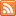# Activity 3: Simulations and Tools CategoryJava applets, interactive simulations, and other statistical tools.

## Applet – Sampling Distribution for a Sample Mean

Published: Feb 12th, 2013

Sampling Distribution Applet: Here is an interactive demonstration which allows you to choose the population, the parameter of interest, and then simulate the sampling distribution of the corresponding statistic for a variety […]

## Learn by Doing – Normal Random Variables

Published: Feb 8th, 2013

The purpose of this interactive activity is to: Get a better feel for the behavior of normal random variables. Check the accuracy of the Standard Deviation Rule. Do some other […]

## Learn by Doing – Shapes of Binomial Distributions

Published: Feb 7th, 2013

Using the online calculator, answer the following questions: Online Binomial Calculator:  Not JAVA Version http://phhp-faculty-cantrell.sites.medinfo.ufl.edu/files/2013/02/qzLBD_08008.swf http://phhp-faculty-cantrell.sites.medinfo.ufl.edu/files/2013/02/qzLBD_08009.swf This document is linked from Binomial Random Variables.

## Learn by Doing – Binomial Probabilities

Published: Feb 3rd, 2013

The purpose of this activity is to give guided practice at solving problems involving binomial random variables and to teach how the same probabilities can be found using an online […]

## Interactive Applet – Conditional Probability

Published: Jan 28th, 2013

Now that we have introduced conditional probability concepts, try this interactive demonstration which uses Venn diagrams to illustrate the probabilities we have been discussing. You can choose which event to shade and […]

## Interactive Applet – Law of Large Numbers

Published: Jan 7th, 2013

This interactive applet is part of the online content for Introduction to the Practice of Statistics, 8th Edition (Moore and McCabe). It allows you to set the true probability and see the Law of Large […]

## Learn By Doing – Relative Frequency

Published: Jan 7th, 2013

Background Recall from the introduction to probability section the birthday problem, which was presented as an example of when probability is counterintuitive. In particular, recall that we asked: “What is […]

## Learn By Doing – Empirical Probability #2

Published: Jan 7th, 2013

Purpose The purpose of this activity is to experiment with an applet to find the P(H) when it is unknown whether the coin is fair or not. The link below […]

## Learn By Doing – Empirical Probability #1

Published: Dec 30th, 2012

Purpose The purpose of this activity is to experiment with an applet that simulates flipping a fair coin, and to see if the P(H) = 0.5. The link below will launch the […]

## Interactive Applet – Let’s Make a Deal

Published: Dec 30th, 2012

Use this applet to gain a feel for this game.  The more trials you conduct the more precise your approximation of this scenario will be! Interactive Applet: Let’s Make A […]